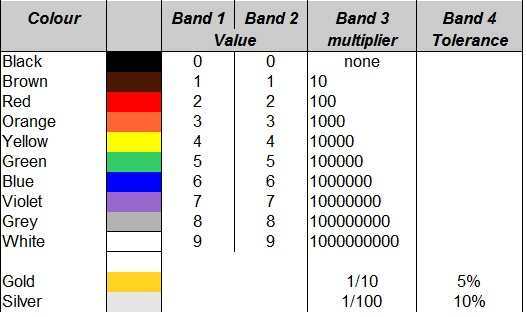# The Resistor Colour Code

By | 05/06/2014

For the intermediate license exam it is necessary to remember and apply the resistor colour code.

The colour code for 5% and 10% resistors
The basic colour code for 5% and 10% tolerance resistors contains 4 colour bands. From left to right the first 3 bands indicate the resistance value. The 4th and last band is the tolerance band.

Band1 and band2 indicate the first two digits of the resistor value. Band3 indicates the multiplier to be applied to the two digits. Multipliers are factors of x10.

Applying the table below to the colour bands on the resistor above shows the following. Bands 1 and 2, Green and Blue provide the first two digits of its value, 5 and 6. Band 3 coloured Black, indicates that there is no multiplier. The 4th and final band, Gold, indicates a tolerance of 5%.  This means that we have a 56 Ohm resistor with a +/- 5% tolerance on its nominal value.Resistor Colour Code

56 Ohm, 5% resistor.
See table and explanation above.

The multiplier band
The multiplier band can sometimes cause confusion. To help clarify the interpretation of the multiplier band several examples are shown below. All the examples have the first two bands set to Green, Blue (5, 6), and the 4th band, tolerance, set to Gold (5%).  The 3rd band, the multiplier, however is shown with many different colours (values).

560 Ohm, 5% resistor.
Moving from a Black 3rd band multiplier on the 56 Ohm resistor , to a Brown band on the resistor below, alters the multiplier to x10. This resistor has a value of 56 x 10 = 560 Ohm.

5600 Ohm, 5% resistor.
Changing the Band 3 multiplier to Red makes the multiplier x100. Why is Red used for x100 when Red is associated with the number 2? The reason is that we are multiplying 10 x 10 to obtain a multiplier of 100. In other words there are two 10’s multiplied together to obtain 100. This is known as raising 10 to the power of 2 and can be written as 10^2 The resistor value is now 56 x 100 = 5600 Ohm ( 5.6 kOhm).

56,000 Ohm ( 5.6 kOhm), 5% resistor
With the third band set to Orange, the multiplier is 10^3 = 10 x 10 x 10= 1000.  Another was to look at this is  to treat Orange (number 3) as an indication of the number of zeros in the multiplier 1000 ( 3 zeros here). This makes the resistor value = 56 x 1000 = 56000 Ohms ( 56 kOhm).

560,000 Ohm ( 560 kOhm), 5% resistor
The third band set to Yellow indicates a multiplier of 10^4 =10 x 10 x 10 x10 = 10000. This makes the resistor value 56 x 10000 = 560000 Ohm ( 560 kOhm).

5,600,000 Ohm ( 5.6 M Ohm), 5% resistor.
With the third band set to Green the multiplier is 10^5 = 10 x 10 x 10 x10 x10 = 100000. This makes the resistance 56 x 100000 = 5600000 Ohm ( 5.6 MOhm).

Resistor Values less than 10 Ohm
From the Resistor colour code (see Table at top) it can be seen that two of the colours normally used to designated % Tolerance ( Gold and Silver) are also used in the multiplier band. These are specifically used for resistor values less than 10 Ohm. In this instance Gold is used to indicated a division of 10  or multiplication by 1/10 th. The Silver band indicates division of 100, or multiplication by 1/100 th.

5.6 Ohm, 5% Resistor
With the third band set to Gold the multiplier is now a fraction of the value, 1 / 10 ( one tenth). This brings the value of this resistor to 56 x 1/10 = 5.6 Ohm.  The fourth band is also Gold and indicates the 5% tolerance as before.

0.56 Ohm, 5% Resistor
With the third band set to Silver the multiplier is now a fraction of the value, 1 / 100  (one hundredth). This brings the value of this resistor to 56 x 1/100 = 0.56 Ohm.  The fourth band is Gold and indicates the 5% tolerance as before.Hopefully this entry has clarified the use of multipliers in the 4 band colour code for 5% and 10% resistors. Only 5% examples were shown here. For 10% tolerance resistors the examples  are still relevant however. The only difference would be in the 4th band coloured Silver to indicate a 10% tolerance in the indicated value, and not the Gold 5% tolerance.

Category: Training